×

Search anything:

#### Algorithms bitwise operationGet this book -> Problems on Array: For Interviews and Competitive Programming

In this article, we have explained how to add any two positive numbers using the bitwise operators like `and`, `xor`, and `left shift` operators rather than using the normal addition operator (`+`).

Table of topics:

1. Basics of Bitwise Operations
2. Adding two numbers using bitwise operators
4. Time and Space Complexity of bitwise add

# Basics of Bitwise Operations

We know that computer stores all kinds of data (videos, files, photos, etc.) in the form of binary numbers `0`s and `1`s. These `0`s and `1`s are called bits and the various operations that can be carried out on these binary numbers are called bitwise operations.

The various bitwise operators are given below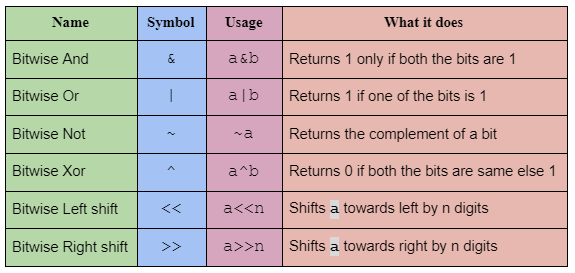Let's take an example and see how each of these operators work.

Consider 2 decimal numbers `a` and `b`.

`a = 25` the binary equivalent of 25 is `00011001`

`b = 14` the binary equivalent of 14 is `00001110`

1. Bitwise And - The Bitwise and returns 1 only if both the bits are 1.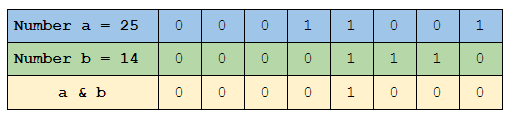2. Bitwise Or - The Bitwise or returns 1 if either of the bits is 1.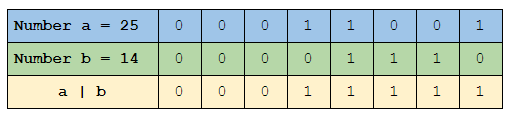3. Bitwise Not - The Bitwise not returns the complement of the bit.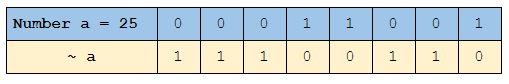4. Bitwise Xor - The Bitwise xor returns 1 only if one of the bits is zero.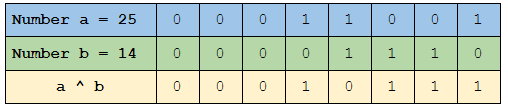5. Bitwise Left Shift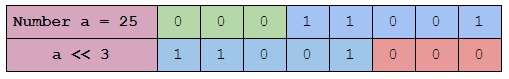In the above image, we can see that three `0`s have been added on the right side i.e. after the Least significant bit, causing the shift towards the left side.

6. Bitwise Right shift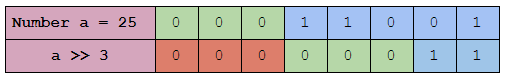In the above image, we can see that three `0`s have been added on the left side i.e. after the Most significant bit, causing the shift towards the right side.

Now since we have got the idea of how the bitwise operators work, let's move on to adding two numbers without the addition operator.

# Adding two numbers using bitwise operators

Let's first take a look at how addition takes place at the binary level and understand it before trying to do it with bitwise operators.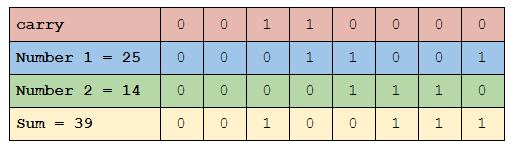The binary addition is pretty similar to usual addition. From the above example, we can understand that

• `1 + 0 = 0 + 1 = 1`
• `0 + 0 = 1`
• `1 + 1 = 10` i.e. the binary equivalent of 2

And another important point to note is that when we get `10`, `1` is taken over to the carry and `0` is kept at the bottom itself.

A truth table will give a better understanding of how the binary addition takes place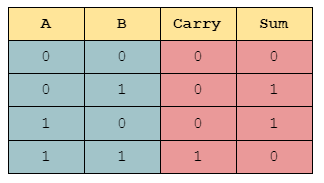From the truth table, we infer that

• The carry expression is `A & B`
• The Sum expression is `A ^ B`

Using the above two expressions the addition of any two numbers can be done as follows.

Steps

1. Get two positive numbers `a` and `b` as input
2. Then checks if the number `b` is not equal to `0`
• Finds the `carry` value (`a & b`)
• Finds the `sum` value (`a ^ b`) and stores it in the variable `a`
• Then shifts the carry to the left by 1-bit stores it in `b`
3. again goes back to step 2
4. When b becomes 0 it finally returns the sum
``````def Bitwise_add(a,b):
while b != 0:
carry = a&b # Carry value is calculated
a = a^b # Sum value is calculated and stored in a
b = carry<<1 # The carry value is shifted towards left by a bit

return a # returns the final sum
``````

.
The whole idea behind this code is that the carry gets added again with the sum value. So what we do is we find the value of the carry separately using the expression `a & b` and use it again to find the sum. We keep on doing this till the carry value becomes 0.

Another important point to note here is that we shift the carry towards the left by 1 bit. That's because the carry value is added to the next bit rather than the current bit. Take a look at this image to get a better understanding.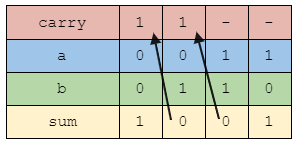We keep on repeating this process till the carry value i.e. `a & b` becomes `0` . And finally, we get the required sum of the two numbers.

Adding the numbers using the bitwise operators can also be done in a recursive manner. The same logic takes place but instead of a loop, we use a recursive function to do the Addition.

``````def Bitwise_add(a,b):
if b == 0:
return a
else :
return Bitwise_add(a^b , (a&b) << 1)
``````

.
In this code, `a^b` is the sum expression, and `(a&b) << 1` is the carry expression after shifting. So it is directly passed as input to the recursive function. And when the carry expression becomes `0` i.e. `b` becomes `0`, the recursion loop stops.

# Time and Space Complexity of bitwise add

The time complexity of the algorithm is O(N), where N is the number of bits in the numbers. The space complexity of the algorithm is O(1).

Given a number M, the number of bits N is equal to logM. So, adding two numbers M is not a constant time O(1) operation. It takes log(M) time. Base of log is 2.

## Conclusion

Bitwise operations are an integral part of any programming language and having a good understanding of them will help you become a better programmer. With this article at OpenGenus, you must have a strong idea of addition using bitwise operation.